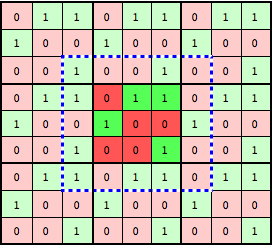The Game of Life is an example of cellular automaton that utilizes a 2 dimensional stencil. For each game iteration, the integer value of each cell in the 2D game grid is determined by summing it’s 8 closest neighbors and then applying the game rules, with the initial game state randomly generated. Each cell has two states, alive or dead, represented as an integer 1 or 0. Periodic boundary conditions are enforced through the use of ghost cells. Cell updates are not propagated through until the end of each iteration, leaving the board static during calculations.

If we wish to play GOL with a 3×3 grid to compensate for the periodic boundary conditions we will need to actually hold a 5×5 grid in memory. This would be the area inside of the blue dashed line in the image below. The cells that are not part of the ‘visible’ 3×3 grid but part of the 5×5 grid we will refer to as ghost cells.## GOL.c

```#include <stdio.h>
#include <stdlib.h>

#define SRAND_VALUE 1985

// Add up all neighbors and return the number of live neighbors
int getNeighbors(int** grid, int i, int j)
{
int numNeighbors;
numNeighbors = grid[i+1][j] + grid[i-1][j]     //lower upper
+ grid[i][j+1] + grid[i][j-1]     //right left
+ grid[i+1][j+1] + grid[i-1][j-1] //diagonals
+ grid[i-1][j+1] + grid[i+1][j-1];

return numNeighbors;
}

int main(int argc, char* argv[])
{
int i,j,iter;
// Linear game grid dimension
int dim = 1024;
// Number of game iterations
int maxIter = 1024;

// Allocate square grid of (dim+2)^2 elements, 2 added for ghost cells
int **grid = (int**) malloc( sizeof(int*) * (dim+2) );
for(i = 0; i<dim+2; i++)
grid[i] = (int*) malloc( sizeof(int*) * (dim+2) );

// Allocate newGrid
int **newGrid = (int**) malloc( sizeof(int*) * (dim+2) );
for(i = 0; i<dim+2; i++)
newGrid[i] = (int*) malloc( sizeof(int*) * (dim+2) );

srand(SRAND_VALUE);
for(i = 1; i<=dim; i++) {
for(j = 1; j<=dim; j++) {
grid[i][j] = rand() % 2;
}
}

// Main game loop
for (iter = 0; iter<maxIter; iter++) {
// Left-Right columns
for (i = 1; i<=dim; i++) {
grid[i] = grid[i][dim]; // Copy first real column to right ghost column
grid[i][dim+1] = grid[i]; // Copy last real column to left ghost column
}
// Top-Bottom rows
for (j = 0; j<=dim+1; j++) {
grid[j] = grid[dim][j]; // Copy first real row to bottom ghost row
grid[dim+1][j] = grid[j]; // Copy last real row to top ghost row
}

// Now we loop over all cells and determine their fate
for (i = 1; i<=dim; i++) {
for (j = 1; j<=dim; j++) {
// Get the number of neighbors for a given grid point
int numNeighbors = getNeighbors(grid, i, j);

// Here we have explicitly all of the game rules
if (grid[i][j] == 1 && numNeighbors < 2)
newGrid[i][j] = 0;
else if (grid[i][j] == 1 && (numNeighbors == 2 || numNeighbors == 3))
newGrid[i][j] = 1;
else if (grid[i][j] == 1 && numNeighbors > 3)
newGrid[i][j] = 0;
else if (grid[i][j] == 0 && numNeighbors == 3)
newGrid[i][j] = 1;
else
newGrid[i][j] = grid[i][j];
}
}

// Done with one step so we swap our grids and iterate again
int **tmpGrid = grid;
grid = newGrid;
newGrid = tmpGrid;
}// End main game loop

// Sum up alive cells and print results
int total = 0;
for (i = 1; i<=dim; i++) {
for (j = 1; j<=dim; j++) {
total += grid[i][j];
}
}
printf("Total Alive: %d\n", total);

// Release memory
free(grid);
free(newGrid);

return 0;
}
```

### Compiling GOL.c

```\$ cc GOL.c -o gol.out
```

### Running GOL.c

```\$ aprun ./gol.out
Total Alive: 45224
```

## GOL.f90

```! Add up all neighbors and return the number of live neighbors
integer function neighbors(grid, i, j)
implicit none
integer,intent(in) :: grid(:,:), i, j
neighbors = grid(i,j+1) + grid(i,j-1)&    !right & left
+ grid(i+1,j) + grid(i-1,j)&    !lower & upper
+ grid(i+1,j+1) + grid(i-1,j-1)& !diagonals
+ grid(i-1,j+1) + grid(i+1,j-1)
return
end function neighbors

program main
implicit none
interface
integer function neighbors(grid, i, j)
integer,intent(in) :: grid(:,:), i, j
end function neighbors
endinterface

integer :: i,j,iter,seed(8),numNeighbors,total
real :: randm
! Linear game grid dimension
integer :: dim = 1024
! Number of game iterations
integer :: maxIter = 2**10

! Game grid pointers
integer,dimension(:,:),pointer :: grid, newGrid, tmpGrid

! Allocate square grid of (dim+2)^2 elements, 2 added for ghost cells
allocate(grid(dim+2,dim+2))
allocate(newGrid(dim+2,dim+2))

seed = (/1985, 2011, 2012, 500, 24, 15, 99, 8/)
call random_seed(PUT=seed)
do j=1,dim
do i=1,dim
call random_number(randm)
grid(i,j) = nint(randm)
enddo
enddo

! Main game loop
do iter=1,maxITer
! Top-Bottom ghost rows
do j=2,dim+1
grid(1,j) = grid(dim+1,j) !Copy first game grid row to bottom ghost row
grid(dim+2,j) = grid(2,j) !Copy first game grid row to top ghost row
enddo

! Left-Right ghost columns
do i=1,dim+2
grid(i,1) = grid(i, dim+1) !Copy first game grid column to right ghost column
grid(i,dim+2) = grid(i,2)  !Copy last game grid column to left ghost column
enddo

! Now we loop over all cells and determine their fate
do j=2,dim+1
do i=2,dim+1
! Get the number of neighbors for a given grid point
numNeighbors = neighbors(grid, i ,j)

! Here we have explicitly all of the game rules
if(grid(i,j) == 1 .AND. numNeighbors < 2) then
newGrid(i,j) = 0
elseif(grid(i,j) == 1 .AND. (numNeighbors == 2 .OR. numNeighbors == 3)) then
newGrid(i,j) = 1
elseif(grid(i,j) == 1 .AND. numNeighbors > 3) then
newGrid(i,j) = 0
elseif(grid(i,j) == 0 .AND. numNeighbors == 3) then
newGrid(i,j) = 1
else
newGrid(i,j) = grid(i,j)
endif
enddo
enddo

! Done with one step so we swap our grids and iterate again
tmpGrid => grid
grid => newGrid
newGrid => tmpGrid

enddo! End main game loop

! Sum up alive cells and print results
total = 0
do j=2,dim+1
do i=2,dim+1
total = total + grid(i,j)
enddo
enddo
print *, "Total Alive", total

! Release memory
deallocate(grid)
deallocate(newGrid)

end program
```

### Compiling GOL.f90

```\$ ftn GOL.f90 -o GOL.out
```

### Running GOL.f90

```\$ aprun ./gol.out
Total Alive        46464
```